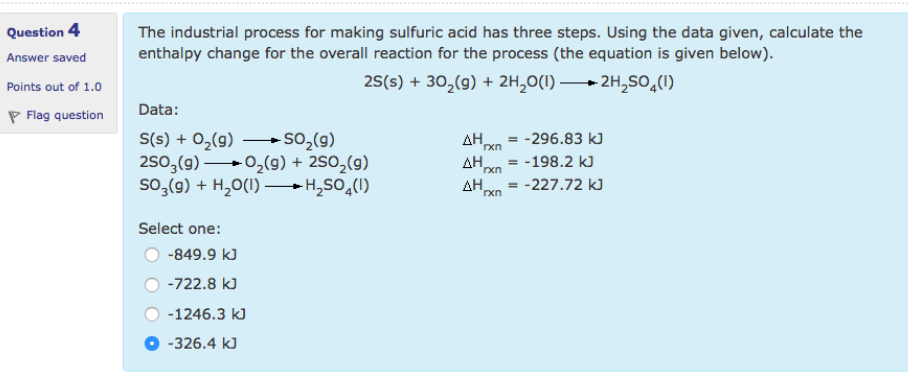# Problem: The industrial process for making sulfuric acid has three steps. Using the data given, calculate the enthalpy change for the overall reaction for the process (the equation is given below).2S(s) + 3O2(g) rightarrow 2H2SO4(l) Data:S(s) + O2(g) → SO(g) Δ Hrxn = -296.83 kJ2SO3(g) → O2(g) + 2SO2(g) ΔHrxn = - 198.2 kJSO3(g) + H2O(l) → H2SO4(l) ΔHrxn = - 227.72 kJSelect one:•-849.9 kJ•-722.8 kJ•-1246.3 kJ•-326.4 kJ

###### FREE Expert Solution
86% (324 ratings)###### Problem Details

The industrial process for making sulfuric acid has three steps. Using the data given, calculate the enthalpy change for the overall reaction for the process (the equation is given below).

2S(s) + 3O2(g) rightarrow 2H2SO4(l)

Data:
S(s) + O2(g) → SO(g) Δ Hrxn = -296.83 kJ
2SO3(g) → O2(g) + 2SO2(g) ΔHrxn = - 198.2 kJ
SO3(g) + H2O(l) → H2SO4(l) ΔHrxn = - 227.72 kJ

Select one:

•-849.9 kJ
•-722.8 kJ
•-1246.3 kJ
•-326.4 kJ